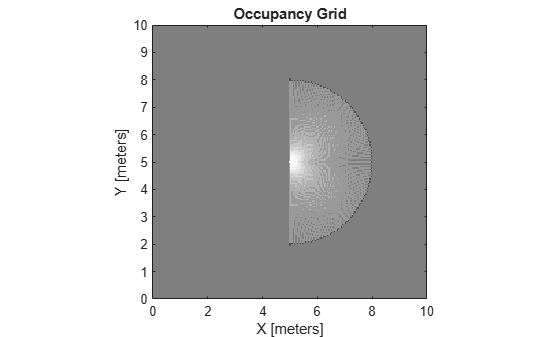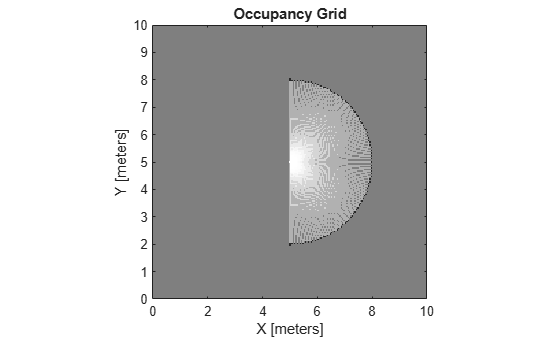getOccupancy

Get occupancy value of locations

Description

example

occVal = getOccupancy(map,xy) returns an array of probability occupancy values at the xy locations in the world frame. Values close to 1 represent a high probability that the cell contains an obstacle. Values close to 0 represent a high probability that the cell is not occupied and obstacle free. Unknown locations, including outside the map, return map.DefaultValue.

occVal = getOccupancy(map,xy,'local') returns an array of occupancy values at the xy locations in the local frame.

occVal = getOccupancy(map,ij,'grid') specifies ij grid cell indices instead of xy locations.

[occVal,validPts] = getOccupancy(___) additionally outputs an n-element vector of logical values indicating whether input coordinates are within the map limits.

occMatrix = getOccupancy(map) returns all occupancy values in the map as a matrix.

occMatrix = getOccupancy(map,bottomLeft,matSize) returns a matrix of occupancy values by specifying the bottom-left corner location in world coordinates and the matrix size in meters.

occMatrix = getOccupancy(map,bottomLeft,matSize,'local') returns a matrix of occupancy values by specifying the bottom-left corner location in local coordinates and the matrix size in meters.

occMatrix = getOccupancy(map,topLeft,matSize,'grid') returns a matrix of occupancy values by specifying the top-left corner location in grid indices and the matrix size.

Examples

collapse all

Create an empty occupancy grid map.

map = occupancyMap(10,10,20);

Input pose of the vehicle, ranges, angles, and the maximum range of the laser scan.

pose = [5,5,0];
ranges = 3*ones(100,1);
angles = linspace(-pi/2,pi/2,100);
maxrange = 20;

Create a lidarScan object with the specified ranges and angles.

scan = lidarScan(ranges,angles);

Insert the laser scan data into the occupancy map.

insertRay(map,pose,scan,maxrange);

Show the map to see the results of inserting the laser scan.

show(map)Check the occupancy of the spot directly in front of the vehicle.

getOccupancy(map,[8 5])
ans = 0.7000

Add a second reading and view the update to the occupancy values. The additional reading increases the confidence in the readings. The free and occupied values become more distinct.

insertRay(map,pose,scan,maxrange);
show(map)getOccupancy(map,[8 5])
ans = 0.8448

Access occupancy values and check their occupancy status based on the occupied and free thresholds of the occupancyMap object.

Create a matrix and populate it with values. Use this matrix to create an occupancy map.

p = 0.5*ones(20,20);
p(11:20,11:20) = 0.75*ones(10,10);
map = occupancyMap(p,10);

Get the occupancy of different locations and check their occupancy statuses. The occupancy status returns 0 for free space and 1 for occupied space. Unknown values return –1.

pocc = getOccupancy(map,[1.5 1])
pocc = 0.7500
occupied = checkOccupancy(map,[1.5 1])
occupied = 1
pocc2 = getOccupancy(map,[5 5],'grid')
pocc2 = 0.5000
occupied2 = checkOccupancy(map,[5 5],'grid')
occupied2 = -1

Input Arguments

collapse all

Map representation, specified as a occupancyMap object. This object represents the environment of the vehicle. The object contains a matrix grid with values representing the probability of the occupancy of that cell. Values close to 1 represent a high probability that the cell contains an obstacle. Values close to 0 represent a high probability that the cell is not occupied and obstacle free.

World coordinates, specified as an n-by-2 matrix of [x y] pairs, where n is the number of world coordinates.

Data Types: double

Grid positions, specified as an n-by-2 matrix of [i j] pairs in [rows cols] format, where n is the number of grid positions.

Data Types: double

Location of bottom left corner of output matrix in world or local coordinates, specified as a two-element vector, [xCoord yCoord]. Location is in world or local coordinates based on syntax.

Data Types: double

Output matrix size, specified as a two-element vector, [xLength yLength] or [gridRow gridCol]. Size is in world, local, or grid coordinates based on syntax.

Data Types: double

Location of top left corner of grid, specified as a two-element vector, [iCoord jCoord].

Data Types: double

Output Arguments

collapse all

Probability occupancy values, returned as a column vector the same length as either xy or ij.

Values close to 0 represent a high probability that the cell is not occupied and obstacle free.

Valid map locations, returned as an n-by-1 column vector equal in length to xy or ij. Locations inside the map return a value of 1. Locations outside the map limits return a value of 0.

Matrix of occupancy values, returned as matrix with size equal to matSize or the size of map.

Values close to 0 represent a high probability that the cell is not occupied and obstacle free.

Limitations

Occupancy values have a limited resolution of ±0.001. The values are stored as int16 using a log-odds representation. This data type limits resolution, but saves memory when storing large maps in MATLAB®. When calling setOccupancy and then getOccupancy, the value returned might not equal the value you set. For more information, see the log-odds representations section in Occupancy Grids.